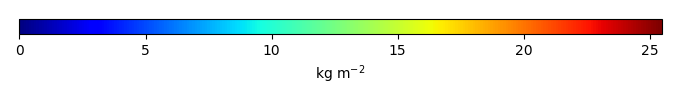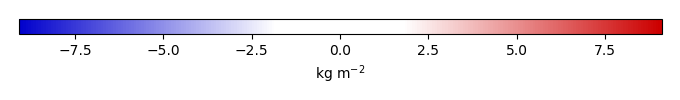# Mean State

Period Mean (original grids) [Pg]
Model Period Mean (intersection) [Pg]
Model Period Mean (complement) [Pg]
Benchmark Period Mean (intersection) [Pg]
Benchmark Period Mean (complement) [Pg]
Bias [kg m-2]
Bias Score 
Spatial Distribution Score 
Overall Score 
Benchmark [-] 32.8
CLM5PHSOFF [-] 47.2 46.4 0.899 32.8 0.0526 3.25 0.60 0.80 0.70
CLM5PHSON [-] 38.5 38.7 0.241 32.8 0.0526 1.73 0.72 0.93 0.82
Period Mean (original grids) [Pg]
Model Period Mean (intersection) [Pg]
Model Period Mean (complement) [Pg]
Benchmark Period Mean (intersection) [Pg]
Benchmark Period Mean (complement) [Pg]
Bias [kg m-2]
Bias Score 
Spatial Distribution Score 
Overall Score 
Benchmark [-] 8.35
CLM5PHSOFF [-] 2.30 1.83 0.177 8.34 0.0114 -1.14 0.43 0.54 0.49
CLM5PHSON [-] 3.24 2.69 0.201 8.34 0.0114 -0.977 0.48 0.62 0.55
Period Mean (original grids) [Pg]
Model Period Mean (intersection) [Pg]
Model Period Mean (complement) [Pg]
Benchmark Period Mean (intersection) [Pg]
Benchmark Period Mean (complement) [Pg]
Bias [kg m-2]
Bias Score 
Spatial Distribution Score 
Overall Score 
Benchmark [-] 10.6
CLM5PHSOFF [-] 10.4 8.62 1.31 10.6 0.0246 -0.228 0.41 0.50 0.45
CLM5PHSON [-] 12.3 11.7 0.363 10.6 0.0246 0.299 0.50 0.56 0.53
Period Mean (original grids) [Pg]
Model Period Mean (intersection) [Pg]
Model Period Mean (complement) [Pg]
Benchmark Period Mean (intersection) [Pg]
Benchmark Period Mean (complement) [Pg]
Bias [kg m-2]
Bias Score 
Spatial Distribution Score 
Overall Score 
Benchmark [-] 4.69
CLM5PHSOFF [-] 3.50 3.38 0.140 4.67 0.0241 -0.393 0.55 0.66 0.60
CLM5PHSON [-] 4.17 4.12 0.0636 4.67 0.0241 -0.0436 0.58 0.80 0.69
Period Mean (original grids) [Pg]
Model Period Mean (intersection) [Pg]
Model Period Mean (complement) [Pg]
Benchmark Period Mean (intersection) [Pg]
Benchmark Period Mean (complement) [Pg]
Bias [kg m-2]
Bias Score 
Spatial Distribution Score 
Overall Score 
Benchmark [-] 27.8
CLM5PHSOFF [-] 78.2 68.2 7.85 26.8 0.921 11.6 0.46 0.89 0.67
CLM5PHSON [-] 39.9 38.2 1.51 26.8 0.921 7.87 0.61 0.92 0.76
Period Mean (original grids) [Pg]
Model Period Mean (intersection) [Pg]
Model Period Mean (complement) [Pg]
Benchmark Period Mean (intersection) [Pg]
Benchmark Period Mean (complement) [Pg]
Bias [kg m-2]
Bias Score 
Spatial Distribution Score 
Overall Score 
Benchmark [-] 43.5
CLM5PHSOFF [-] 32.7 31.7 0.504 43.5 0.0288 -1.30 0.45 0.61 0.53
CLM5PHSON [-] 52.5 51.4 0.326 43.5 0.0288 1.03 0.49 0.63 0.56
Period Mean (original grids) [Pg]
Model Period Mean (intersection) [Pg]
Model Period Mean (complement) [Pg]
Benchmark Period Mean (intersection) [Pg]
Benchmark Period Mean (complement) [Pg]
Bias [kg m-2]
Bias Score 
Spatial Distribution Score 
Overall Score 
Benchmark [-] 32.0
CLM5PHSOFF [-] 27.8 25.6 2.06 31.9 0.0804 -0.408 0.57 0.85 0.71
CLM5PHSON [-] 31.6 31.8 0.632 31.9 0.0804 0.262 0.61 0.86 0.73
Period Mean (original grids) [Pg]
Model Period Mean (intersection) [Pg]
Model Period Mean (complement) [Pg]
Benchmark Period Mean (intersection) [Pg]
Benchmark Period Mean (complement) [Pg]
Bias [kg m-2]
Bias Score 
Spatial Distribution Score 
Overall Score 
Benchmark [-] 21.1
CLM5PHSOFF [-] 32.8 28.1 2.82 20.9 0.146 1.00 0.52 0.71 0.62
CLM5PHSON [-] 24.8 23.3 0.610 20.9 0.146 1.28 0.60 0.82 0.71
Period Mean (original grids) [Pg]
Model Period Mean (intersection) [Pg]
Model Period Mean (complement) [Pg]
Benchmark Period Mean (intersection) [Pg]
Benchmark Period Mean (complement) [Pg]
Bias [kg m-2]
Bias Score 
Spatial Distribution Score 
Overall Score 
Benchmark [-] 3.81
CLM5PHSOFF [-] 5.61 5.80 0.158 3.81 0.000319 0.574 0.53 0.78 0.66
CLM5PHSON [-] 6.88 7.17 0.0941 3.81 0.000319 1.03 0.51 0.75 0.63
Period Mean (original grids) [Pg]
Model Period Mean (intersection) [Pg]
Model Period Mean (complement) [Pg]
Benchmark Period Mean (intersection) [Pg]
Benchmark Period Mean (complement) [Pg]
Bias [kg m-2]
Bias Score 
Spatial Distribution Score 
Overall Score 
Benchmark [-] 9.16
CLM5PHSOFF [-] 18.3 14.3 3.29 8.87 0.289 0.648 0.59 0.78 0.68
CLM5PHSON [-] 11.0 10.0 0.829 8.87 0.289 0.760 0.60 0.81 0.71
Period Mean (original grids) [Pg]
Model Period Mean (intersection) [Pg]
Model Period Mean (complement) [Pg]
Benchmark Period Mean (intersection) [Pg]
Benchmark Period Mean (complement) [Pg]
Bias [kg m-2]
Bias Score 
Spatial Distribution Score 
Overall Score 
Benchmark [-] 12.0
CLM5PHSOFF [-] 21.3 18.6 2.16 12.0 0.0529 1.14 0.42 0.35 0.39
CLM5PHSON [-] 18.2 17.4 0.537 12.0 0.0529 1.77 0.47 0.35 0.41
Period Mean (original grids) [Pg]
Model Period Mean (intersection) [Pg]
Model Period Mean (complement) [Pg]
Benchmark Period Mean (intersection) [Pg]
Benchmark Period Mean (complement) [Pg]
Bias [kg m-2]
Bias Score 
Spatial Distribution Score 
Overall Score 
Benchmark [-] 368.
CLM5PHSOFF [-] 542. 500. 43.1 363. 4.64 1.09 0.50 0.70 0.60
CLM5PHSON [-] 487. 477. 9.77 363. 4.64 1.46 0.57 0.78 0.68
Period Mean (original grids) [Pg]
Model Period Mean (intersection) [Pg]
Model Period Mean (complement) [Pg]
Benchmark Period Mean (intersection) [Pg]
Benchmark Period Mean (complement) [Pg]
Bias [kg m-2]
Bias Score 
Spatial Distribution Score 
Overall Score 
Benchmark [-] 1.24
CLM5PHSOFF [-] 0.978 0.599 0.280 1.23 0.00783 -0.137 0.42 0.66 0.54
CLM5PHSON [-] 1.55 1.22 0.341 1.23 0.00783 0.0659 0.51 0.74 0.62
Period Mean (original grids) [Pg]
Model Period Mean (intersection) [Pg]
Model Period Mean (complement) [Pg]
Benchmark Period Mean (intersection) [Pg]
Benchmark Period Mean (complement) [Pg]
Bias [kg m-2]
Bias Score 
Spatial Distribution Score 
Overall Score 
Benchmark [-] 5.62
CLM5PHSOFF [-] 11.6 10.9 0.500 5.59 0.0265 0.893 0.45 0.48 0.46
CLM5PHSON [-] 14.5 14.1 0.228 5.59 0.0265 1.60 0.34 0.40 0.37
Period Mean (original grids) [Pg]
Model Period Mean (intersection) [Pg]
Model Period Mean (complement) [Pg]
Benchmark Period Mean (intersection) [Pg]
Benchmark Period Mean (complement) [Pg]
Bias [kg m-2]
Bias Score 
Spatial Distribution Score 
Overall Score 
Benchmark [-] 8.21
CLM5PHSOFF [-] 16.3 13.8 1.71 8.09 0.122 1.71 0.46 0.48 0.47
CLM5PHSON [-] 10.2 9.46 0.474 8.09 0.122 1.74 0.54 0.60 0.57
Period Mean (original grids) [Pg]
Model Period Mean (intersection) [Pg]
Model Period Mean (complement) [Pg]
Benchmark Period Mean (intersection) [Pg]
Benchmark Period Mean (complement) [Pg]
Bias [kg m-2]
Bias Score 
Spatial Distribution Score 
Overall Score 
Benchmark [-] 14.8
CLM5PHSOFF [-] 7.11 5.93 0.824 14.8 0.0147 -2.14 0.50 0.77 0.63
CLM5PHSON [-] 12.0 11.7 0.182 14.8 0.0147 -0.425 0.65 0.87 0.76
Period Mean (original grids) [Pg]
Model Period Mean (intersection) [Pg]
Model Period Mean (complement) [Pg]
Benchmark Period Mean (intersection) [Pg]
Benchmark Period Mean (complement) [Pg]
Bias [kg m-2]
Bias Score 
Spatial Distribution Score 
Overall Score 
Benchmark [-] 20.1
CLM5PHSOFF [-] 32.6 31.8 0.793 19.8 0.219 2.14 0.45 0.65 0.55
CLM5PHSON [-] 43.6 43.6 0.247 19.8 0.219 4.91 0.40 0.72 0.56
Period Mean (original grids) [Pg]
Model Period Mean (intersection) [Pg]
Model Period Mean (complement) [Pg]
Benchmark Period Mean (intersection) [Pg]
Benchmark Period Mean (complement) [Pg]
Bias [kg m-2]
Bias Score 
Spatial Distribution Score 
Overall Score 
Benchmark [-] 8.04
CLM5PHSOFF [-] 1.45 1.37 0.182 7.99 0.0515 -1.66 0.41 0.25 0.33
CLM5PHSON [-] 3.04 3.04 0.0352 7.99 0.0515 -1.10 0.51 0.49 0.50
Period Mean (original grids) [Pg]
Model Period Mean (intersection) [Pg]
Model Period Mean (complement) [Pg]
Benchmark Period Mean (intersection) [Pg]
Benchmark Period Mean (complement) [Pg]
Bias [kg m-2]
Bias Score 
Spatial Distribution Score 
Overall Score 
Benchmark [-] 88.9
CLM5PHSOFF [-] 150. 147. 1.87 88.8 0.0655 6.82 0.53 0.78 0.66
CLM5PHSON [-] 129. 128. 0.373 88.8 0.0655 5.00 0.65 0.94 0.80
Period Mean (original grids) [Pg]
Model Period Mean (intersection) [Pg]
Model Period Mean (complement) [Pg]
Benchmark Period Mean (intersection) [Pg]
Benchmark Period Mean (complement) [Pg]
Bias [kg m-2]
Bias Score 
Spatial Distribution Score 
Overall Score 
Benchmark [-] 9.09
CLM5PHSOFF [-] 20.5 19.6 1.02 9.08 0.0128 1.50 0.35 0.26 0.30
CLM5PHSON [-] 21.8 21.8 0.283 9.08 0.0128 2.13 0.32 0.30 0.31
Period Mean (original grids) [Pg]
Model Period Mean (intersection) [Pg]
Model Period Mean (complement) [Pg]
Benchmark Period Mean (intersection) [Pg]
Benchmark Period Mean (complement) [Pg]
Bias [kg m-2]
Bias Score 
Spatial Distribution Score 
Overall Score 
Benchmark [-] 2.45
CLM5PHSOFF [-] 8.11 7.87 0.449 2.40 0.0496 1.82 0.37 0.27 0.32
CLM5PHSON [-] 6.26 6.15 0.140 2.40 0.0496 1.92 0.35 0.32 0.34

# Temporally integrated period mean

BENCHMARK MEANMODEL MEANBIASBIAS SCORESPATIAL TAYLOR DIAGRAMMODEL COLORS# Spatially integrated regional mean

MODEL COLORS# All Models

BenchmarkCLM5PHSOFFCLM5PHSON